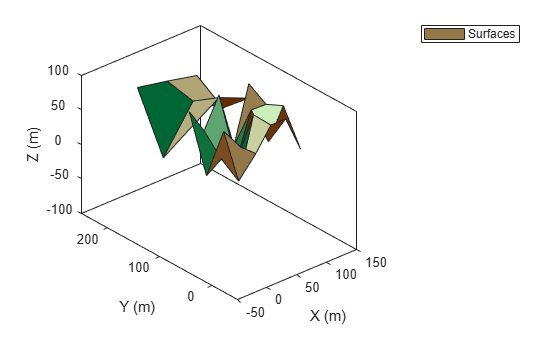# plotSurface

Plot surfaces in theater surface plotter

Since R2022b

## Syntax

``plotSurface(sPlotter,plotData)``

## Description

example

````plotSurface(sPlotter,plotData)` plots surfaces specified by `plotData` using the surface plotter `sPlotter`.```

## Examples

collapse all

Create a tracking scenario.

`scene = trackingScenario;`

Define the terrain and boundaries of two surfaces and add the two surfaces to the tracking scenario.

```terrain1 = randi(100,4,5); terrain2 = randi(100,3,3); boundary1 = [0 100; 0 100-eps]; boundary2 = [0 100; 100 200]; s1 = groundSurface(scene,Terrain=terrain1,Boundary=boundary1); s2 = groundSurface(scene,Terrain=terrain2,Boundary=boundary2);```

Obtain the plotter data by using the `surfacePlotterData` function.

`plotterData = surfacePlotterData(scene.SurfaceManager)`
```plotterData=1×2 struct array with fields: X Y Z C ```

Create a `theaterPlot` object and specify its axial limits.

`tp = theaterPlot(ZLimits=[-50 150],YLimits=[-50 250],ZLimits=[-100 100]);`

Create a surface plotter.

`splotter = surfacePlotter(tp,DisplayName="Surfaces");`

Plot surfaces in the theater plot. Change the view angles for better visualization.

```plotSurface(splotter,plotterData) view(-41,29)```## Input Arguments

collapse all

Surface plotter object, created by the `surfacePlotter` function.

Plot data, specified as an S-element array of structures, where S is the number of surfaces. You can directly create this argument by using the `surfacePlotterData` function. To create this argument manually, specify each structure with these fields.

Field NameDescription
`X`Domain of the surface in the x-direction, specified as an M-element real-valued vector. M is the number of x-coordinates for defining the terrain of the surface. The values for the elements in the vector must monotonically increase.
`Y`Domain of the surface in the y-direction, specified as an N-element real-valued vector. N is the number of y-coordinates for defining the terrain of the surface. The values for the elements in the vector must monotonically increase.
`Z`Height values of the surface, specified as an N-by-M real-valued matrix. N is the number of elements in the `Y` field, and M is the number of elements in the X field.
`C`Color for vertices in the terrain of the surface, specified as an N-by-M-by-3 matrix of RGB triplets. N is the number of elements in the `Y` field, and M is the number of elements in the X field. The `plotSurface` function determines the color of a surface patch based on the color of its first vertex.

## Version History

Introduced in R2022b